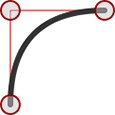# How to Draw Quadratic Curves on an HTML5 Canvas

Drawing graphical curves within the browser has never been easy. Until recently, if you wanted a smooth chart, you either had to generate an image or create an SVG on the server. Creating a curve on the fly required ninja JavaScript skills, a mathematics degree and immense patience.

The canvas element has changed everything. We can now draw and animate complex lines, curves and shapes with a few lines of code. Today we’ll look at quadratic curves.

It’s been a long time since I took a mathematics lesson, so please don’t expect an in-depth explanation! If you’re interested, take a look at the migraine-inducing equations at WolframMathWorldLike any line, a quadratic curve has a start (P0) and end point (P2). It then has a single control point (P1) which determines the curvature of the line. This image from Wikipedia provides a good curve generation illustration.Quadratic curves are great for drawing smooth edges. As you can see from this image, it’s easy to specify a control point where the squared edge would normally appear.

## Enough math — let’s see some code!

The canvas element supports quadratic curves. First, we require a little initialization to get the canvas context and set the default line width and color:

``````
canvas = document.getElementById("canvas");
ctx = canvas.getContext("2d")
ctx.lineWidth = 6;
ctx.strokeStyle = "#333";
``````

We now define the starting point for our curve (P0):

``````
ctx.beginPath();
ctx.moveTo(100, 250);
``````

The quadraticCurveTo() method can now be used to draw a quadratic curve. It takes four arguments:

• the x co-ordinate of the control point (P1)
• the y co-ordinate of the control point (P1)
• the x co-ordinate of the end point (P2)
• the y co-ordinate of the end point (P2)

Finally, we call the stroke() method to complete our curve:

``````